# Polytope examples in morphometer

## Random polygon

This method simulates a random polygon. We choose random angles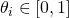until. The coordinates of points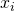are then given by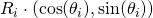where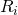are independent random radii between 0 and 150.
(Note that the intervals of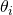andare arbitrarily chosen.)

## Uniform distribution of points on the unit sphere

This method simulates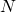random points on the unit sphere by samplingrandom angles theta in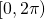. The coordinates of points are then given by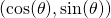. The parameterdefines the number of points and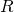the radius of the sphere.

## Random beta polygon

This method simulates a beta polygon created byindependent identically distributed random points with density

(1)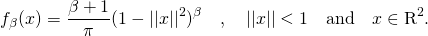For more details, see https://arxiv.org/pdf/1805.01338.pdf.

Here a short description of the simulation of the model:
* Choose the number of points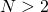* Choose a parameter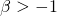* Samplerandom angles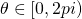* Sampleradii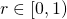that follow the probability density: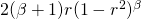by using the inverse transform sampling:
– simply draw a random number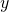uniformly from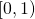– then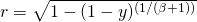* Coordinates of points are then given by r*(cos(theta), sin(theta))